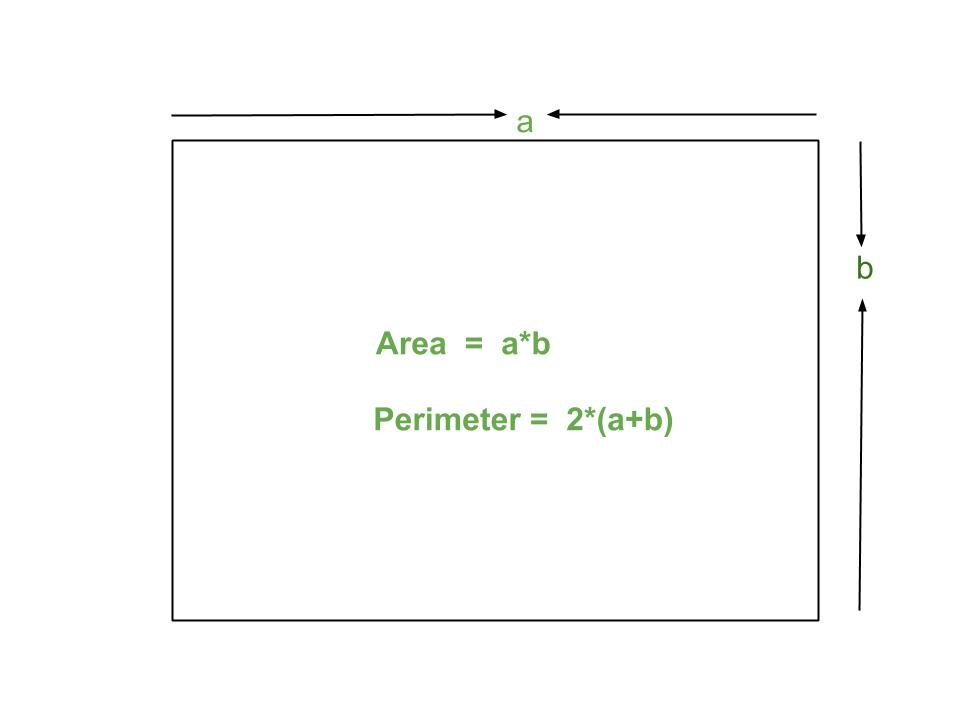GeeksforGeeks App
Open AppBrowser
Continue

# JavaScript program to find Area and Perimeter of Rectangle

A rectangle is a flat figure in a plane. It has four sides and four equal angles of 90 degrees each. In a rectangle all four sides are not of equal length like a square, sides opposite to each other have equal length. Both diagonals of the rectangle have equal lengths.Examples:

```Input: 4 5
Output: Area = 20
Perimeter = 18

Input: 2 3
Output: Area = 6
Perimeter = 10```

Formulae:

```Area of rectangle: a*b
Perimeter of rectangle: 2*(a + b) ```

Example: Below is the example that will illustrate the above formula:

## Javascript

 ``

Output:

```Area = 30
Perimeter = 22```

Time Complexity: O(1) as it is performing constant operations
Auxiliary Space: O(1)

My Personal Notes arrow_drop_up